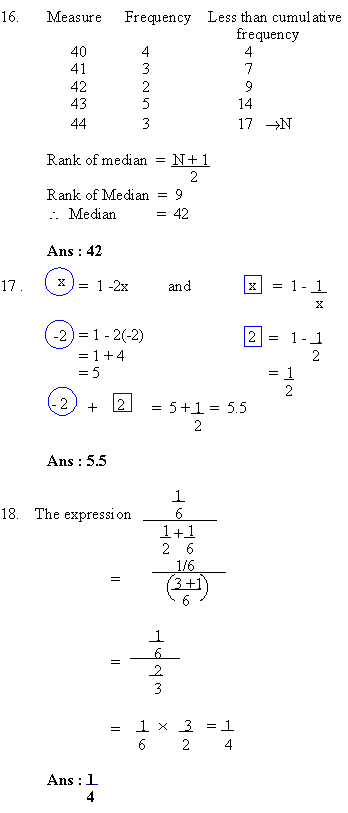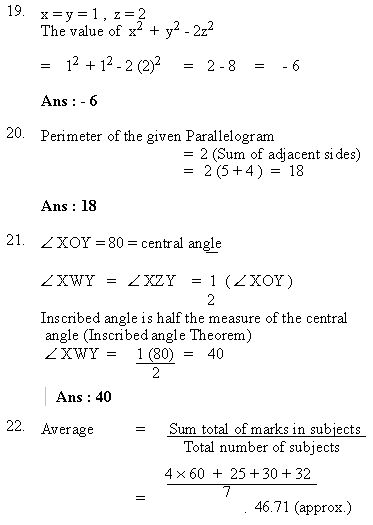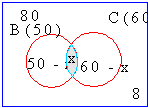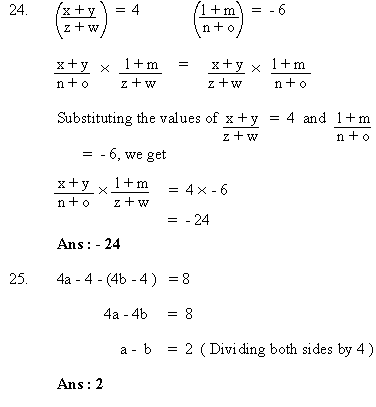Support the Monkey! Tell All your Friends and TeachersHome MonkeyNotes Printable Notes Digital Library Study Guides Study Smart Parents Tips College Planning Test Prep Fun Zone Help / FAQ How to Cite New Title Request

 Part II : Student-Produced Response QuestionsSolving the Venn-Diagram. Let x be the number of students who passed in Biology and Chemistry in senior class at Townsville High school. 80 = (50 - x) + x + (60 - x) + 8 where (50 - x) is the number of students passing in Biology alone. (60 - x) is the number of students passing in Chemistry alone. Solving the equation, we get x = 118 - 80 x = 38 Ans : 38Index Test 1 Section 1 : Verbal Reasoning Section 2 : Mathematical Reasoning Section 3 : Verbal Reasoning Section 4 : Mathematical Reasoning Section 5 : Reading Comprehension Section 6 : Mathematical Reasoning Section 7 : Mathematical Reasoning Answer Key To Test 1 Answer Explanation To Test 1 Section 1 : Verbal Reasoning Section 2 : Mathematical Reasoning Section 3 : Verbal Reasoning Section 4 : Mathematical Reasoning Section 5 : Reading Comprehension Section 6 : Mathematical Reasoning Section 7 : Mathematical Reasoning Test 2
 Search: All Products Books Popular Music Classical Music Video DVD Toys & Games Electronics Software Tools & Hardware Outdoor Living Kitchen & Housewares Camera & Photo Cell Phones Keywords: